Question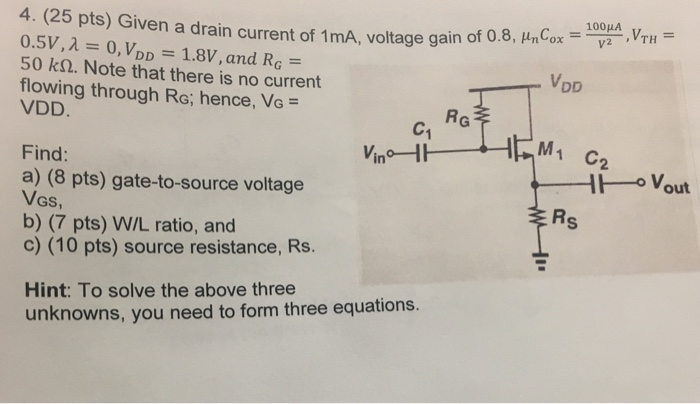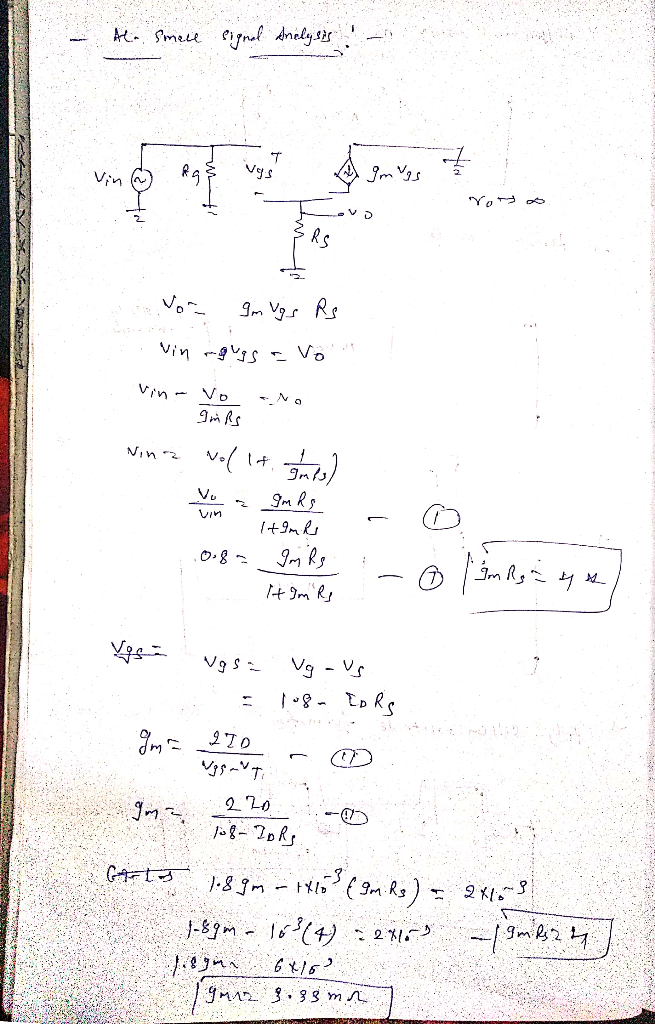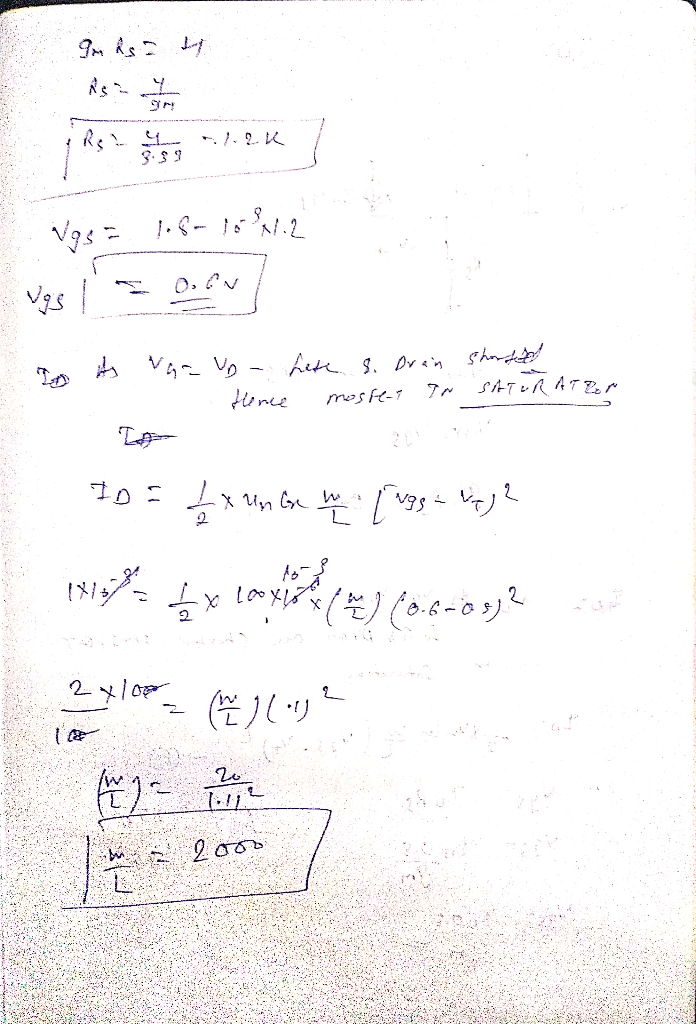#### Earn Coins

Coins can be redeemed for fabulous gifts.

Similar Homework Help Questions
• ### D 6.112 The MOSFET in the circuit of Fig. P6.112 has = 5 mA/V, and V, = 40 V. (a) Find the values of Rs,RD, and RG so that ID=0.4 mA, the largest possible value for R is used while a maximum sig...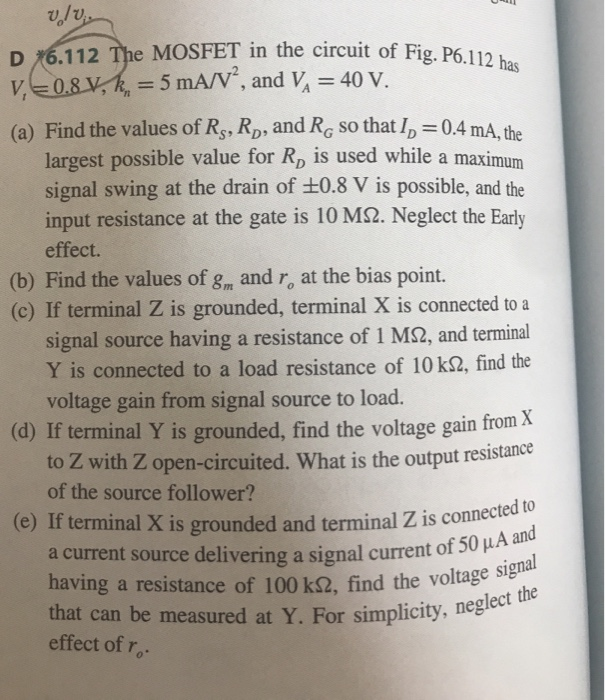D 6.112 The MOSFET in the circuit of Fig. P6.112 has = 5 mA/V, and V, = 40 V. (a) Find the values of Rs,RD, and RG so that ID=0.4 mA, the largest possible value for R is used while a maximum signal swing at the drain of +0.8 V is possible, and the input resistance at the gate is 10 MS2. Neglect the Early effect. (b) Find the values of gm and r, at the bias point. (c) If...

• ### D-17.121 The MOSFET in the circuit of Fig. P7.121 has V, 0.8 V, k,-5 mA/V", and...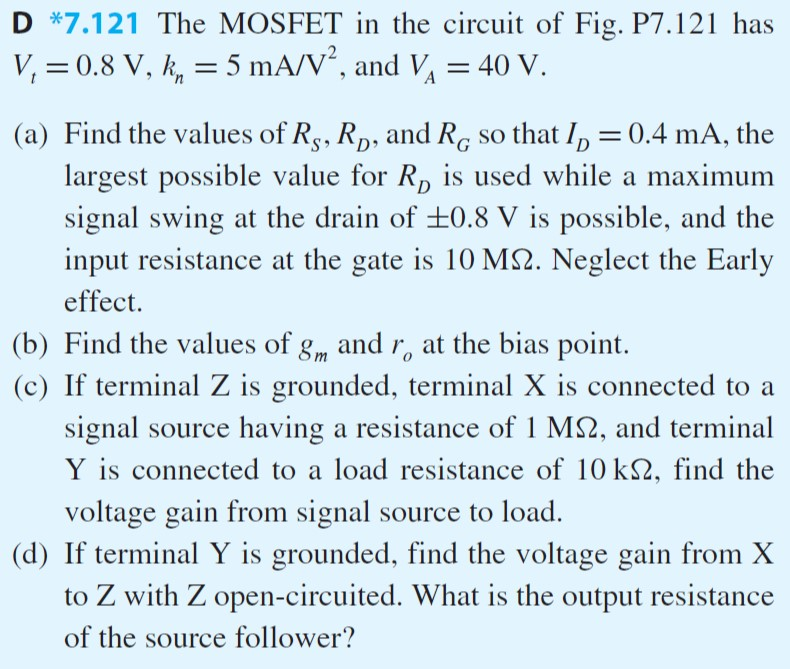D-17.121 The MOSFET in the circuit of Fig. P7.121 has V, 0.8 V, k,-5 mA/V", and V,-40 V (a) Find the values of Rş, Rp, and Ro so that Ip 0.4 mA, the largest possible value for Rp is used while a maximum signal swing at the drain of ±0.8 V is possible, and the input resistance at the gate is 10 M2. Neglect the Early effect. (b) Find the values of g and r at the bias point. (c)...

• ### 3. Consider the following multistage amplifier. The current source values are 1, -2mA, I, - 1mA,...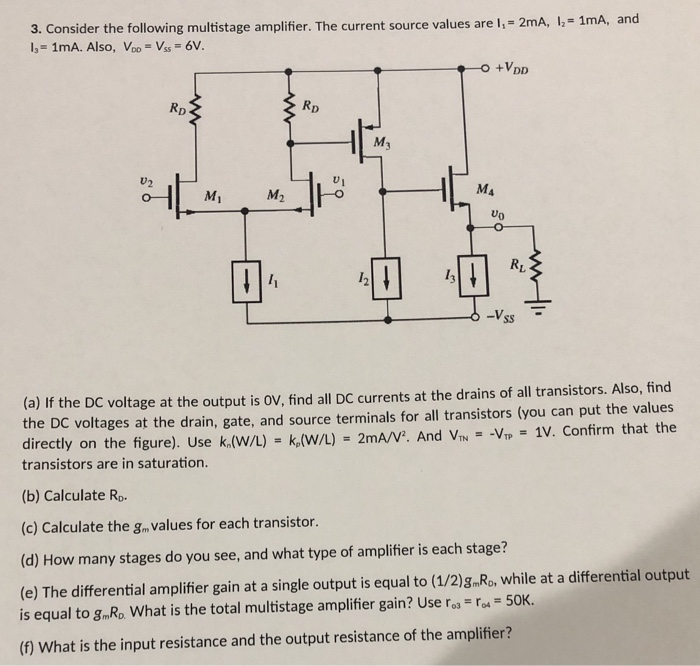3. Consider the following multistage amplifier. The current source values are 1, -2mA, I, - 1mA, and 1 - 1mA. Also, Voo - Vss - 6V. +VDD (a) If the DC voltage at the output is OV, find all DC currents at the drains of all transistors. Also, find the DC voltages at the drain, gate, and source terminals for all transistors (you can put the values directly on the figure). Use k.(W/L) - k.(W/L) = 2mA/V. And Vin -...

• ### Help with completing the table please?? MPF102 Table 8-1 Listed Measured Value Value Resistor Drain Source...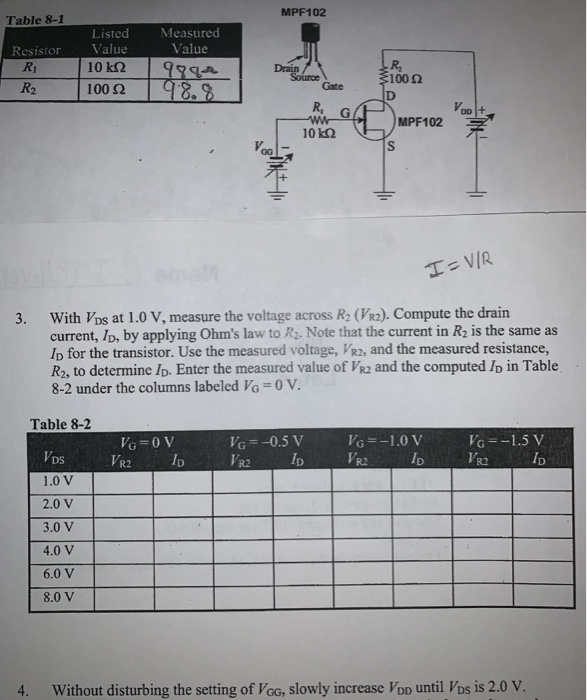Help with completing the table please?? MPF102 Table 8-1 Listed Measured Value Value Resistor Drain Source Gate R1 10 kQ 1000 R2 100 2 D R. wWr 10 k2 Уро MPF102 H= VIR With Vps at 1.0 V, measure the voltage across R2 (VR2). Compute the drain current, ID, by applying Ohm's law to R2. Note that the current in R2 is the same as Ip for the transistor. Use the measured voltage, VR2, and the measured resistance, R2, to...

• ### electronics question #3 (5 pts.) A BJT amplifier has the voltage gain of 80, absolute voltage gain of 60, current gain of 40, and source resistance of 1 k2. Find the load resistance (no need to kn...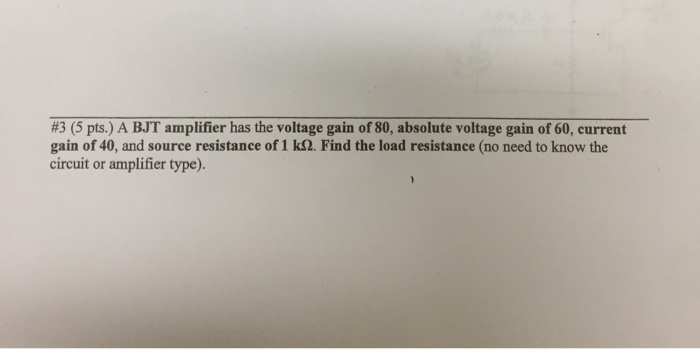electronics question #3 (5 pts.) A BJT amplifier has the voltage gain of 80, absolute voltage gain of 60, current gain of 40, and source resistance of 1 k2. Find the load resistance (no need to know the circuit or amplifier type). #3 (5 pts.) A BJT amplifier has the voltage gain of 80, absolute voltage gain of 60, current gain of 40, and source resistance of 1 k2. Find the load resistance (no need to know the circuit or...

• ### 4. The MOSFET in the circuit given below has Vi- 1 V, kn 0.8 mA/V2, and VA 40 V a) Find the values of Rs, Ro, and Ro so that Io -0.1 mA, the largest possible value for RD is used while a maximum...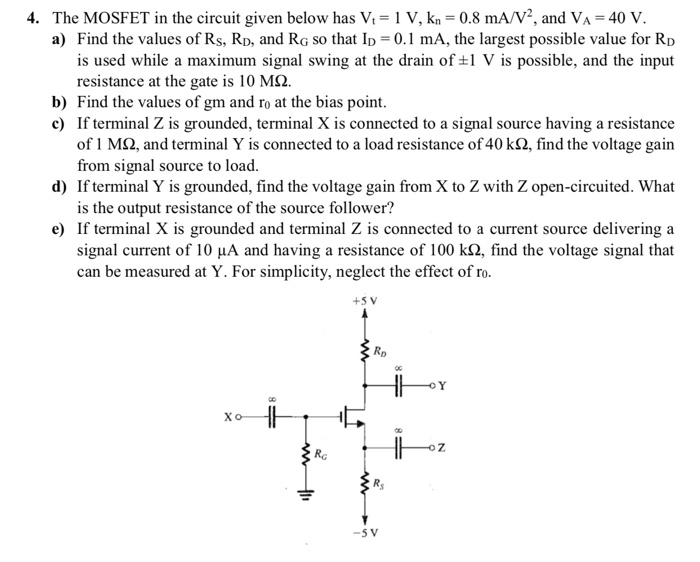4. The MOSFET in the circuit given below has Vi- 1 V, kn 0.8 mA/V2, and VA 40 V a) Find the values of Rs, Ro, and Ro so that Io -0.1 mA, the largest possible value for RD is used while a maximum signal swing at the drain of tl V is possible, and the input resistance at the gate is 10 MS2. b) Find the values of gm and ro at the bias point c) If terminal Z...

• ### Q2 MOSFET and I-V Curves (Total 30 pts) Q2.1 Consider the band diagrams (conduction band) of...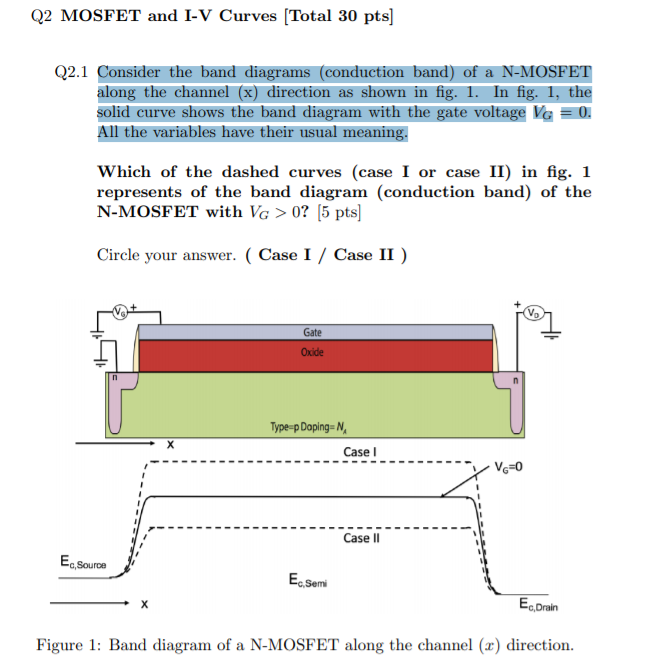Q2 MOSFET and I-V Curves (Total 30 pts) Q2.1 Consider the band diagrams (conduction band) of a N-MOSFET along the channel (x) direction as shown in fig. 1. In fig. 1, the solid curve shows the band diagram with the gate voltage VG = 0. All the variables have their usual meaning. Which of the dashed curves (case I or case II) in fig. 1 represents of the band diagram (conduction band) of the N-MOSFET with VG >0? 5 pts...

• ### 3) CS amplifier shown below is biased by a constant-current source I. Let Rsig 0.5 M, RG -2 MQ, gm -3mA/V, Rp -20 k2, and RL 10 k2. Find the midband voltage gain and the lower 3-dB frequency with...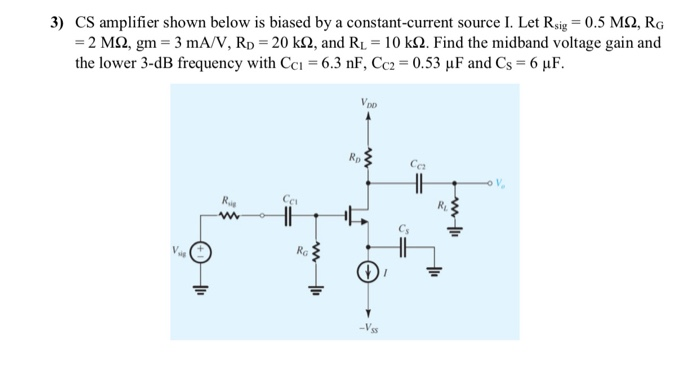3) CS amplifier shown below is biased by a constant-current source I. Let Rsig 0.5 M, RG -2 MQ, gm -3mA/V, Rp -20 k2, and RL 10 k2. Find the midband voltage gain and the lower 3-dB frequency with Cc 6.3 nF, Cc2-0.53 uF and Cs-6 uF. Rp C2 C F 3) CS amplifier shown below is biased by a constant-current source I. Let Rsig 0.5 M, RG -2 MQ, gm -3mA/V, Rp -20 k2, and RL 10 k2. Find...

• ### 6.108 (d) Section 6.5: Discrete-Circuit Amplifiers 6.107 Calculate overall voltage gain G, of a 3 mA/V, ro= = 1...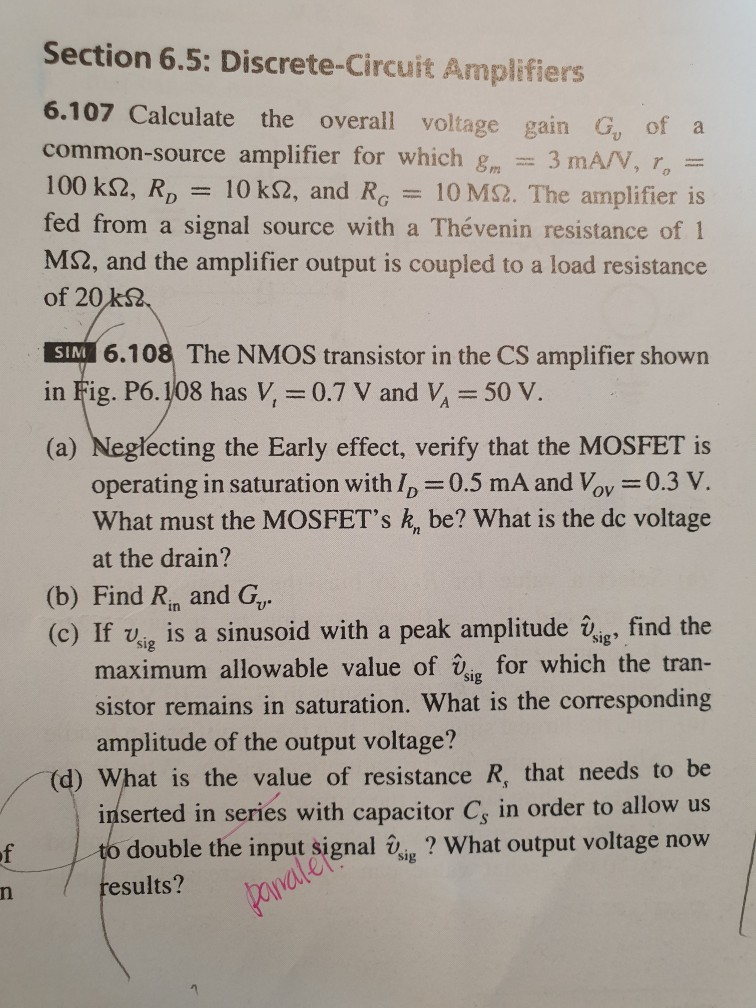6.108 (d) Section 6.5: Discrete-Circuit Amplifiers 6.107 Calculate overall voltage gain G, of a 3 mA/V, ro= = 10 MS2. The amplifier is the common-source amplifier for which g 100 k2, RD 10 k2, and RG fed from a signal source with a Thevenin resistance of l M2, and the amplifier output is coupled of 20 k2 to a load resistance SIM 6.108 The NMOS transistor in the CS amplifier shown in Fig. P6.108 has V, = 0.7 V and...

• ### 5) Consider the Cascode amplifier shown below. For the NMOS transistors, kn 0.2 mA/V2, Vr,-0.5 V,...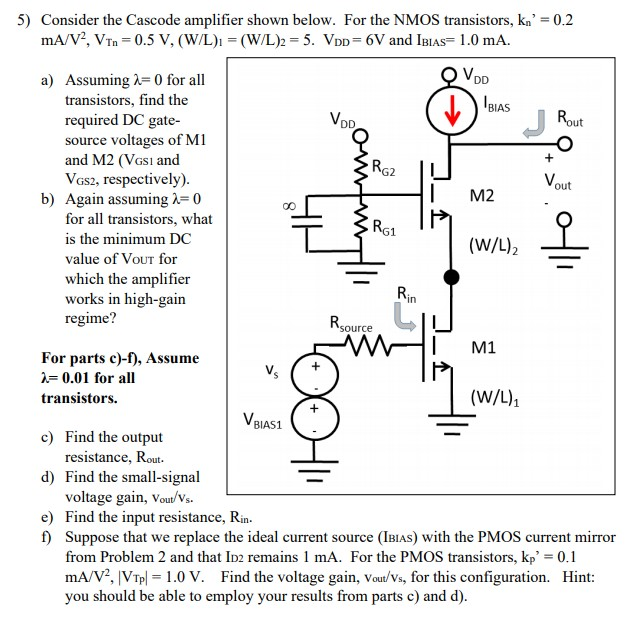5) Consider the Cascode amplifier shown below. For the NMOS transistors, kn 0.2 mA/V2, Vr,-0.5 V, (W/L)-(W/L)2-5. VDD-GV and IBIAs= 1.0 mA. a) Assuming λ-0 for all transistors, find the required DC gate- source voltages of M1 and M2 (VGsı and VGs2, respectively) BIAS VD out b) Again assuming 0 M2 for all transistors, what is the minimum DC value of VouT for which the amplifier works in high-gain regime? (W/L)2 in M1 For parts c)-f), Assume -0.01 for all...